# Two infinitely long, straight wires are parallel and separated by a distance of one meter. They...

Two infinitely long, straight wires are parallel and separated by a distance of one meter. They carry currents in the same direction. Wire 1 carries 6 times thecurrent as wire 2 does. Determine the perpendicular distance from wire 1 to a point where the net magnetic field is zero between the two wires.

Let point p lies between the wires at which net magnetic field is zero

magnetic field at a point P which is at a distance r from the first wire B_1 = μ_0 I / 2πr

magnetic field at a point P due to second wire which is at a distance 1-r from the second wire

B_2 =μ_0*6 I / 2π(1 -r)

From the given condition

B_1 = B_2

μ_0 I / 2πr =μ_0*6 I / 2π(1 -r)

solving for r we get

r = 1/7 m = 0.142 m

#### Earn Coin

Coins can be redeemed for fabulous gifts.

Similar Homework Help Questions
• ### Two infinitely long, straight wires are parallel and separated by a distance of one meter. They...

Two infinitely long, straight wires are parallel and separated by a distance of one meter. They carry currents in the same direction. Wire 1 carries 6 times the current as wire 2 does. Determine the perpendicular distance from wire 1 to a point where the net magnetic field is zero between the two wires.

• ### Two long, straight, parallel wires separated by a distance d carry currents in opposite directions as...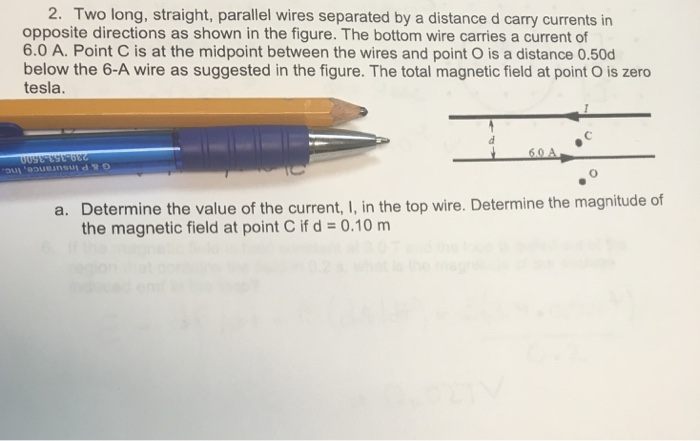Two long, straight, parallel wires separated by a distance d carry currents in opposite directions as shown in the figure. The bottom wire carries a current of 6.0 A. Point C is at the midpoint between the wires and point O is a distance 0.50d below the 6-A wire as suggested in the figure. The total magnetic field at point O is zero tesla. a. Determine the value of the current, I, in the top wire. Determine the magnitude of...

• ### 3) Two parallel infinitely-long wires separated by 10 cm distance carry the currents of 1 A...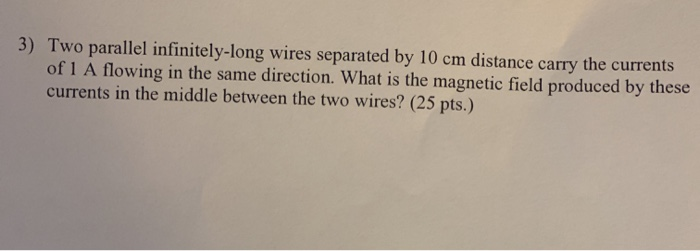3) Two parallel infinitely-long wires separated by 10 cm distance carry the currents of 1 A flowing in the same direction. What is the magnetic field produced by these currents in the middle between the two wires? (25 pts.)

• ### Two long, straight wires separated by a distance of 27.7 cm carry currents in the same...

Two long, straight wires separated by a distance of 27.7 cm carry currents in the same direction. The current in wire 1 is 1.69 A, and the current in wire 2 is 4.29 A. Find the magnitude of the magnetic field at a point midway between the wires.

• ### 2. (1 point) Two infinitely long vertical wires are separated by a distance of 1m. One...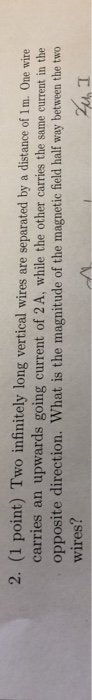2. (1 point) Two infinitely long vertical wires are separated by a distance of 1m. One wire carries an upwards going current of 2 A, while the other carries the same current in the opposite direction. What is the magnitude of the magnetic field half way between the two wires? 2h, I

• ### Two infinitely long, straight parallel wires perpendicular to the plane of the page carry currents of...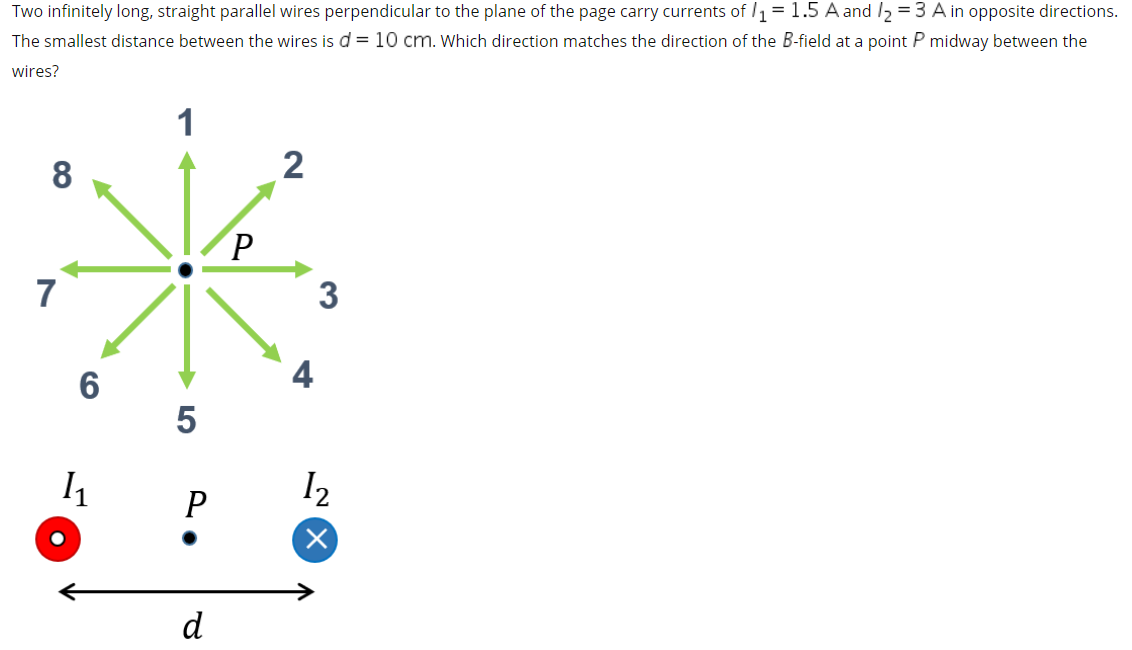Two infinitely long, straight parallel wires perpendicular to the plane of the page carry currents of l1 = 1.5 A and I2 = 3 A in opposite directions. The smallest distance between the wires is d = 10 cm. Which direction matches the direction of the B-field at a point P midway between the wires?

• ### Two long, parallel wires are separated by a distance of , = 4 cm, as shown...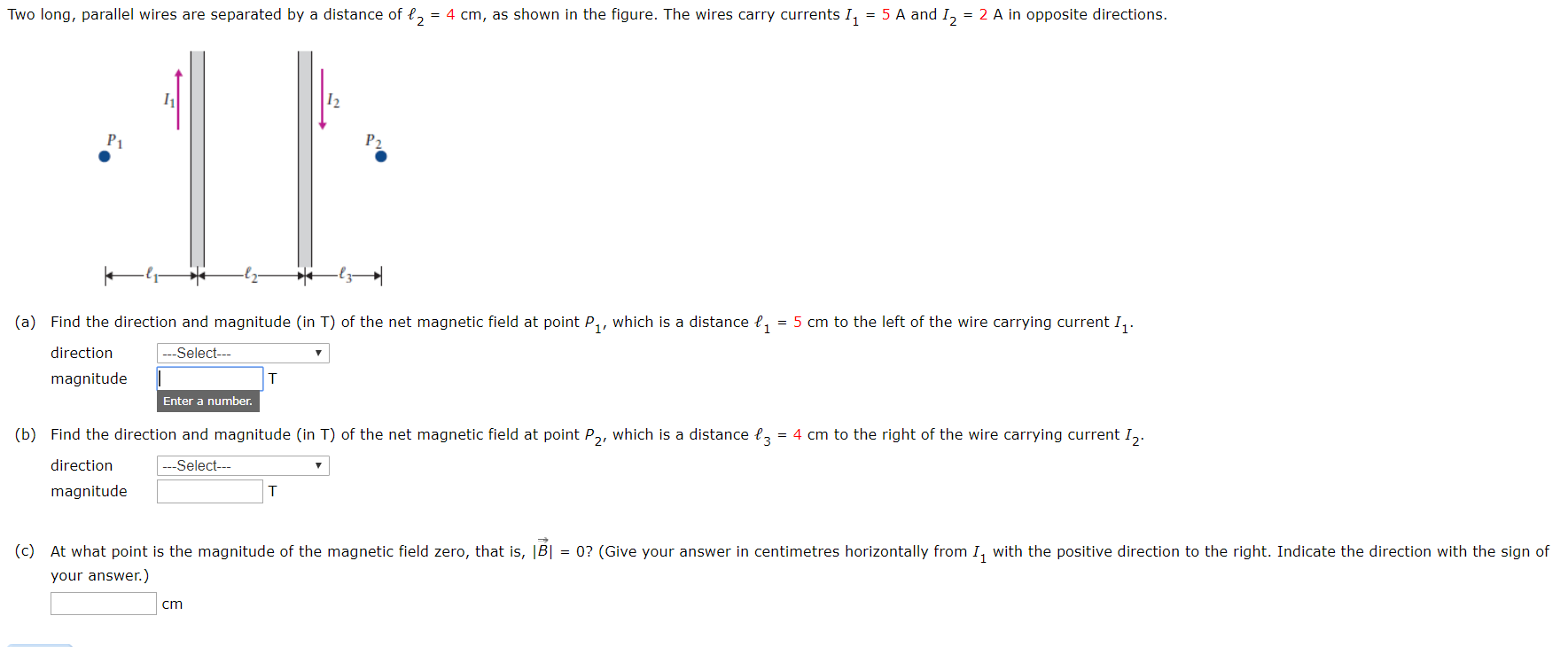Two long, parallel wires are separated by a distance of , = 4 cm, as shown in the figure. The wires carry currents I, = 5 A and I, = 2 A in opposite directions. Р1 Р2 (a) Find the direction and magnitude (in T) of the net magnetic field at point P,, which is a distance , = 5 cm to the left of the wire carrying current I. direction -Select- magnitude T Enter a number. (b) Find the...

• ### Two long straight parallel wires, separated by d1 = 0.775 cm, are perpendicular to the plane...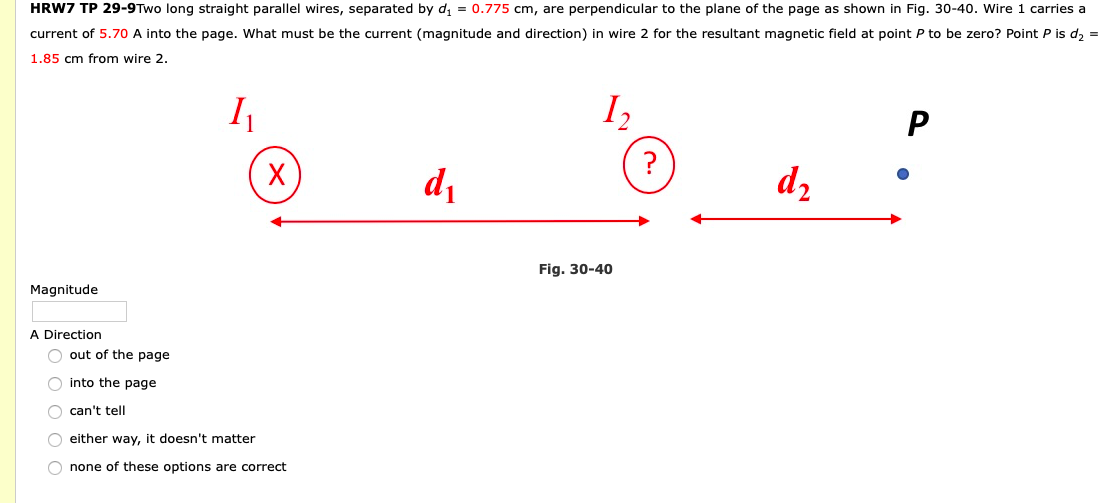Two long straight parallel wires, separated by d1 = 0.775 cm, are perpendicular to the plane of the page as shown in Fig. 30-40. Wire 1 carries a current of 5.70 A into the page. What must be the current (magnitude and direction) in wire 2 for the resultant magnetic field at point P to be zero? Point P is d2 = 1.85 cm from wire 2. HRW7 TP 29-9Two long straight parallel wires, separated by dy = 0.775 cm,...

• ### In a physics experiment, two straight parallel wires are separated by a distance of 1.1 cm....

In a physics experiment, two straight parallel wires are separated by a distance of 1.1 cm. They are both 2.1 meters long and they carry currents in the same direction. Wire 1 carries a current of 13.5 amps and wire 2 carries a current of 15.1 amps. They exert a weak attractive force between them. Calculate the magnitude of that force in millinewtons (mN).

• ### 2. Two long straight parallel wires separated by a distance of 20 cm carry currents of...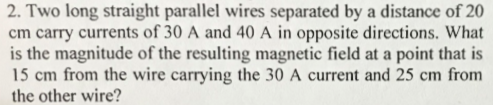2. Two long straight parallel wires separated by a distance of 20 cm carry currents of 30 A and 40 A in opposite directions. What is the magnitude of the resulting magnetic field at a point that is 15 cm from the wire carrying the 30 A current and 25 cm from the other wire?